Area Of Cube/ Cuboid

Chapter 13 Class 9 Surface Areas and Volumes
Concept wise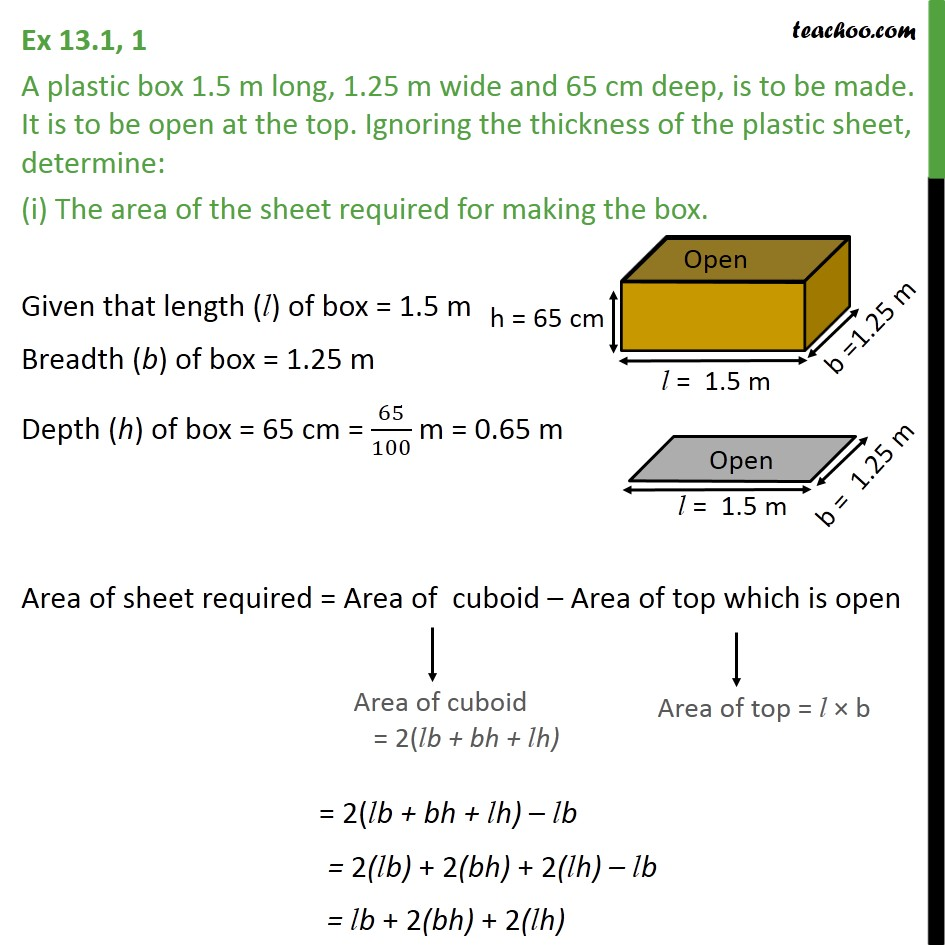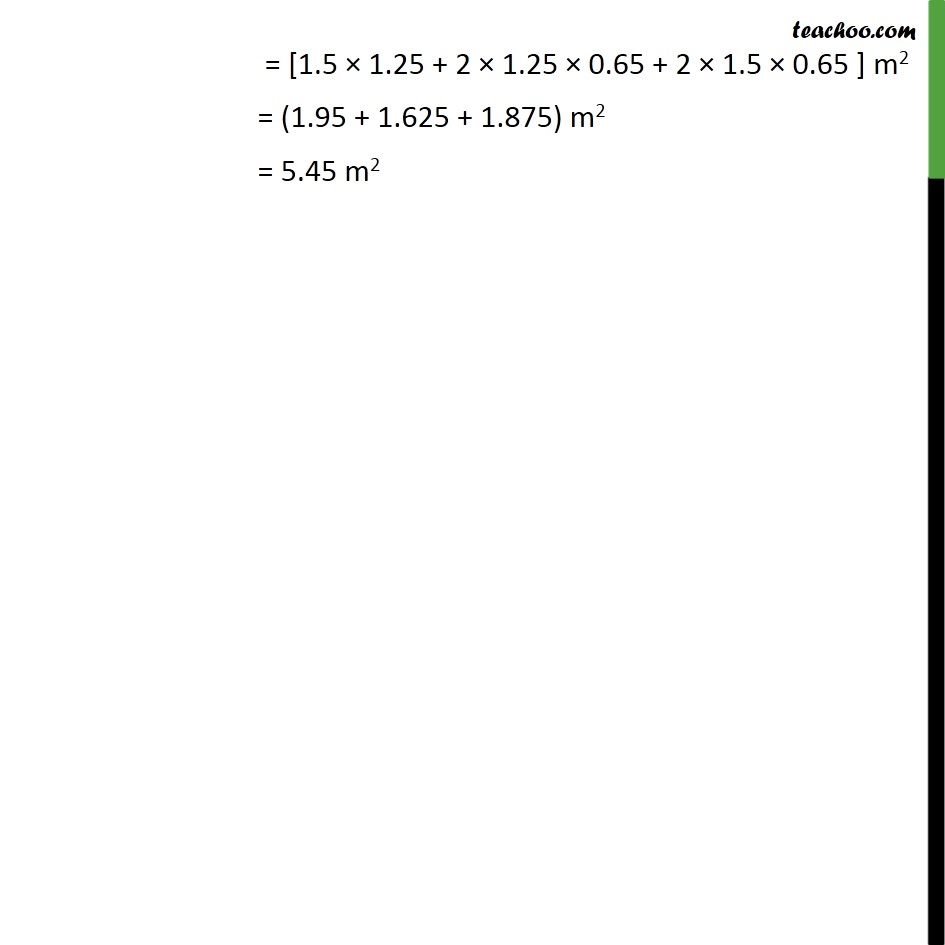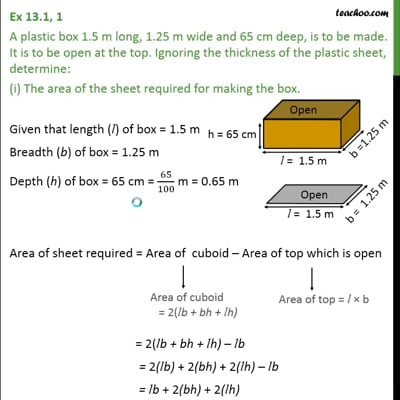This video is only available for Teachoo black users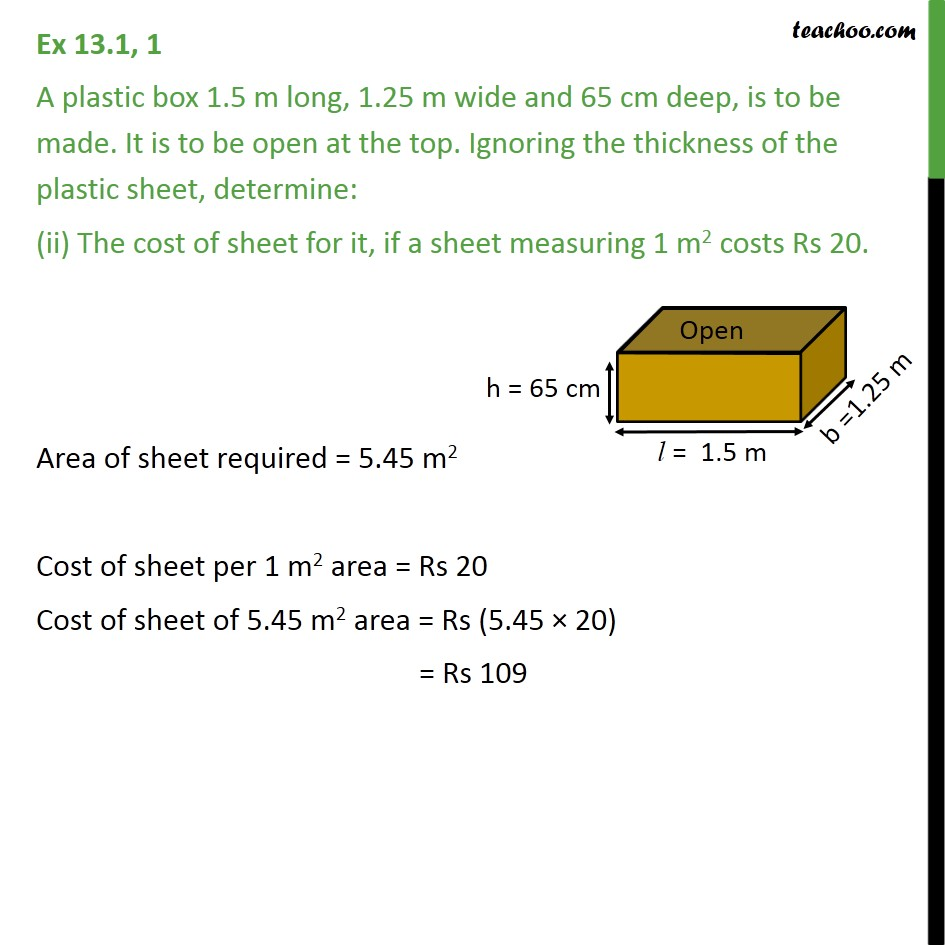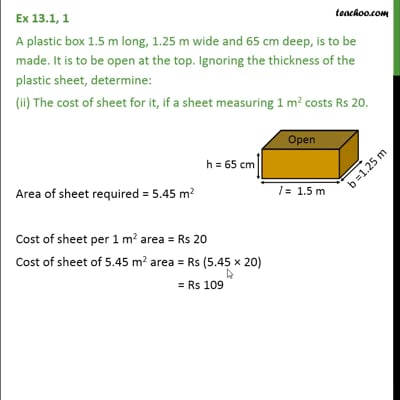This video is only available for Teachoo black users

Introducing your new favourite teacher - Teachoo Black, at only ₹83 per month

### Transcript

Ex 13.1, 1 A plastic box 1.5 m long, 1.25 m wide and 65 cm deep, is to be made. It is to be open at the top. Ignoring the thickness of the plastic sheet, determine: (i) The area of the sheet required for making the box. Given that length (l) of box = 1.5 m Breadth (b) of box = 1.25 m Depth (h) of box = 65 cm = 65/100 m = 0.65 m Area of sheet required = Area of cuboid Area of top which is open = 2(lb + bh + lh) lb = 2(lb) + 2(bh) + 2(lh) lb = lb + 2(bh) + 2(lh) = [1.5 1.25 + 2 1.25 0.65 + 2 1.5 0.65 ] m2 = (1.95 + 1.625 + 1.875) m2 = 5.45 m2 Ex 13.1, 1 A plastic box 1.5 m long, 1.25 m wide and 65 cm deep, is to be made. It is to be open at the top. Ignoring the thickness of the plastic sheet, determine: (ii) The cost of sheet for it, if a sheet measuring 1 m2 costs Rs 20. Area of sheet required = 5.45 m2 Cost of sheet per 1 m2 area = Rs 20 Cost of sheet of 5.45 m2 area = Rs (5.45 20) = Rs 109